Learning Library

Spell the Secret Word: Practice Subtraction

Here's a clever way to mix math with mystery. Can your kid crack the code?

Click on a worksheet in the set below to see more info or download the PDF.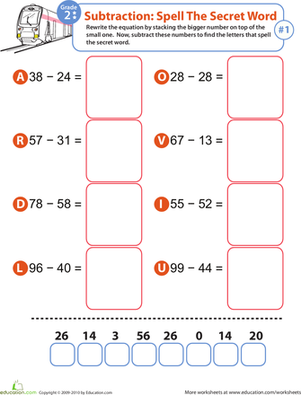Math Riddles: Subtraction

In this second grade math worksheet, children practice writing and solving two-digit subtraction problems. At the end, they'll uncover a secret word.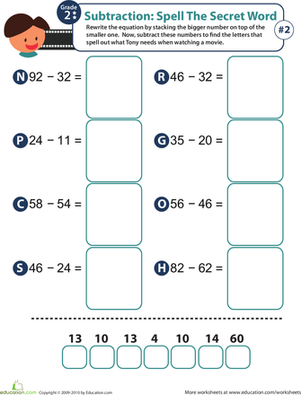Spell the Secret Word: Practice Subtraction 2

Kids practice two-digit subtraction then use their answers to uncover a secret word in this 2nd grade math worksheet.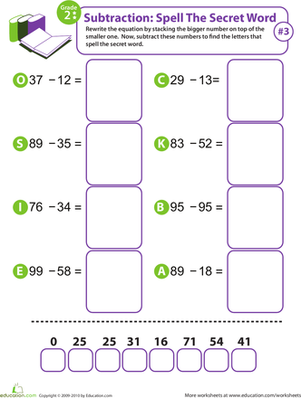Spell the Secret Word: Practice Subtraction 3

Children practice two-digit subtraction and writing equations in this 2nd grade math worksheet. At the end, they'll use their answers to uncover a secret word.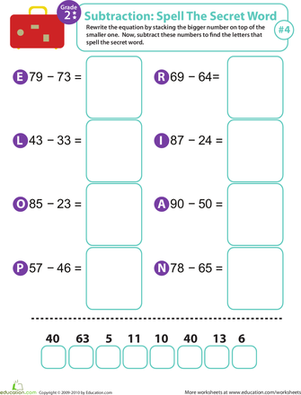Spell the Secret Word: Practice Subtraction 4

Give your child's two-digit subtraction skills a boost with this second grade math worksheet. At the end, they'll use their answers to uncover a secret word.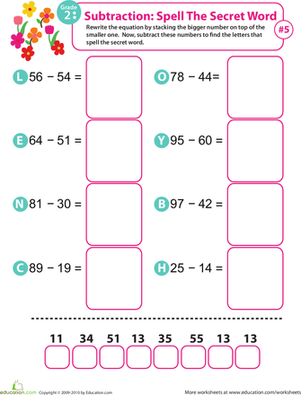Spell the Secret Word: Practice Subtraction 5

Part math practice, part word puzzle, this worksheet makes solving subtraction problems fun. Complete two-digit subtraction problems to reveal a secret word.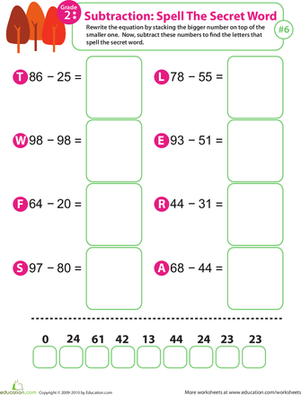Spell the Secret Word: Practice Subtraction 6

In this 2nd grade math worksheet, your child will practice writing equations and subtracting, then use their answers to uncover a secret word.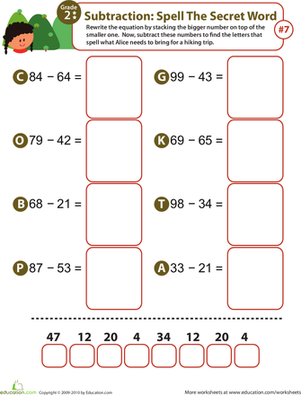Spell the Secret Word: Practice Subtraction 7

Help your kids learn their subtraction facts with this 2nd grade math worksheet. At the end, they'll use their answers to uncover a secret word.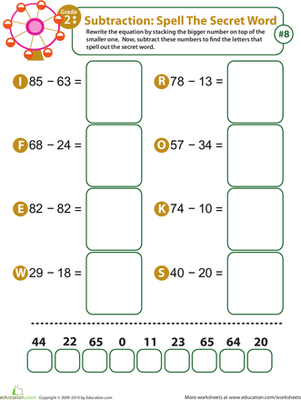Spell the Secret Word: Practice Subtraction 8

In this 2nd grade math worksheet, your child will practice solving subtraction problems, then use their answers to solve the word puzzle at the end.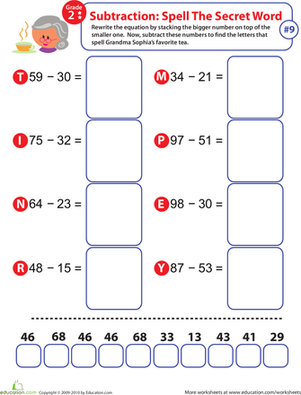Spell the Secret Word: Practice Subtraction 9

Improve your child's subtraction skill with this second grade math worksheet that includes a special word puzzle at the end.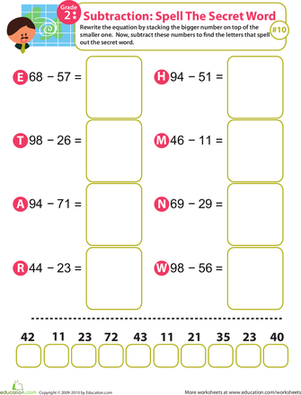Spell the Secret Word: Practice Subtraction 10

This second grade math worksheet makes subtraction practice fun. After completing the problems, your child will use their answers to uncover a secret word.

Want to download this whole set as a single PDF?

Create new collection

0

New Collection>

0 items

What could we do to improve Education.com?

Please note: Use the Contact Us link at the bottom of our website for account-specific questions or issues.

What would make you love Education.com?